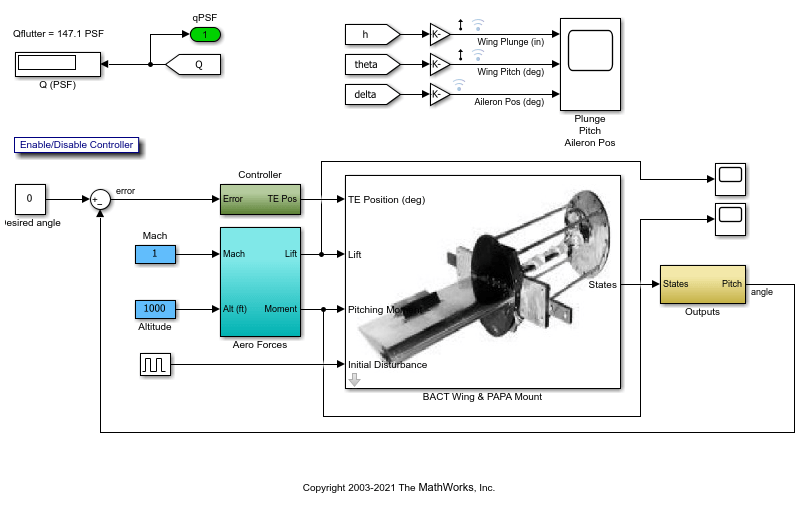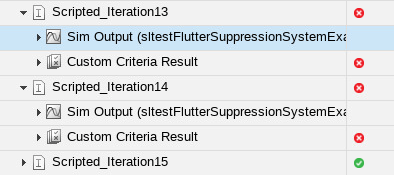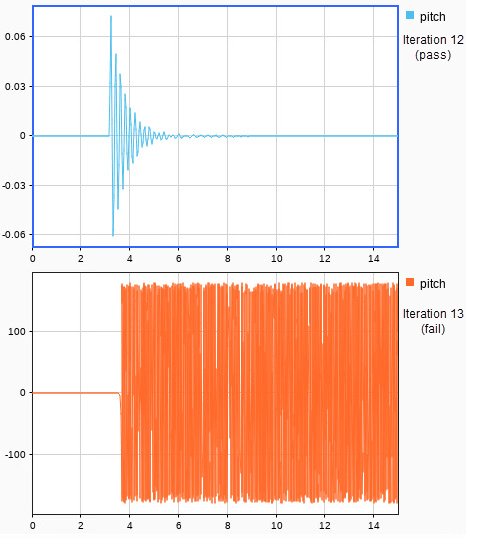# Assess the Damping Ratio of a Flutter Suppression System

This example shows how to use a custom criteria script to verify that wing oscillations are damped in multiple altitude and airspeed conditions.

### The Test and Model

The model uses Simscape™ to simulate a Benchmark Active Controls Technology (BACT) / Pitch and Plunge Apparatus (PAPA) setup. It uses Aerospace Blockset™ to simulate aerodynamic forces on the wing.

The test iterates over 16 combinations of `Mach` and `Altitude`. The test case uses custom criteria with Curve Fitting Toolbox™ to find the peaks of the wing pitch, and determine the damping ratio. If the damping ratio is not greater than zero, the assessment fails.

Click Open Script to open the test file.

```open('sltestFlutterCriteriaTest.mldatx') ```

In the Test Browser, select Altitude and mach iterations. Open the model by clicking the arrow next to Model in the System Under Test section.

```open_system('sltestFlutterSuppressionSystemExample.slx') ```### Custom Criteria Script

The test case custom criteria uses this script to verify that the damping ratio is greater than zero.

```% Get time and data for pitch Time = test.sltest_simout.get('sigsOut').get('pitch').Values.Time(1:15000); Data = test.sltest_simout.get('sigsOut').get('pitch').Values.Data(1:15000); ```
```% Find peaks [~, peakIds] = findpeaks(Data,'minpeakheight', 0.002, 'minpeakdistance', 50); peakTime= Time(peakIds); peakPos = Data(peakIds); rn = peakPos(1)./peakPos(2:end); L = 1:length(rn); ```
```% Do curve fitting fittedModel = exponentialFitAndPlot(L, rn); delta = fittedModel.d; ```
```% Find damping ratio dRatio = delta/sqrt((2*pi)^2+delta^2); ```
```% Make sure damping ratio is greater than 0 test.verifyGreaterThan(dRatio,0,'Damping ratio must be greater than 0'); ```

### Test Results

Running the test case returns two conditions in which the damping ratio is greater than zero.

```results = sltest.testmanager.run; ```The wing pitch plots from iteration 12 and 13 show the difference between a positive damping ratio (iteration 12) and a negative damping ratio (iteration 13).```sltest.testmanager.close close_system('sltestFlutterSuppressionSystemExample.slx',0) ```